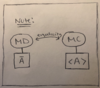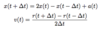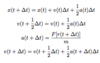# Chapter 1: MD Methods Flashcards Preview

## Simulation Methods I > Chapter 1: MD Methods > Flashcards

Flashcards in Chapter 1: MD Methods Deck (11):
1

2

## Ergodicity Hypothesis

### For significant number of particles, the time average is the same as the ensemble average3

## Ergodicity, MD, MC4

## Overview (MD Methods)

### approximate solutions to equation of motion using finite difference method5

## Euler Method  (Algorithm)

### Derive by Taylor expanding x(t + ∆t )6

7

## Verlet Integrator  (Algorithm)

### derive by Taylor expanding r (t + ∆t ) and r (t - ∆t ) and adding together velocity comes from centered finite difference method8

9

## Velocity-Verlet Integrator  (Algorithm)

### Initial setup Calculate new position x(t + ∆t ) Calculate half-step velocity v(t + 0.5∆t ) Calculate new force a(t + ∆t ) Claculate final velocity v(t + ∆t )10

11